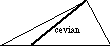index: click on a letter A B C D E F G H I J K L M N O P Q R S T U V W X Y Z A to Z index index: subject areas numbers & symbols sets, logic, proofs geometry algebra trigonometry advanced algebra & pre-calculus calculus advanced topics probability & statistics real world applications multimedia entrieswww.mathwords.com about mathwords website feedback

 Cevian A line segment, ray, or line that extends from a vertex of a triangle to the opposite side (which may be extended). Medians, altitudes, and angle bisectors are all examples of cevians.See also